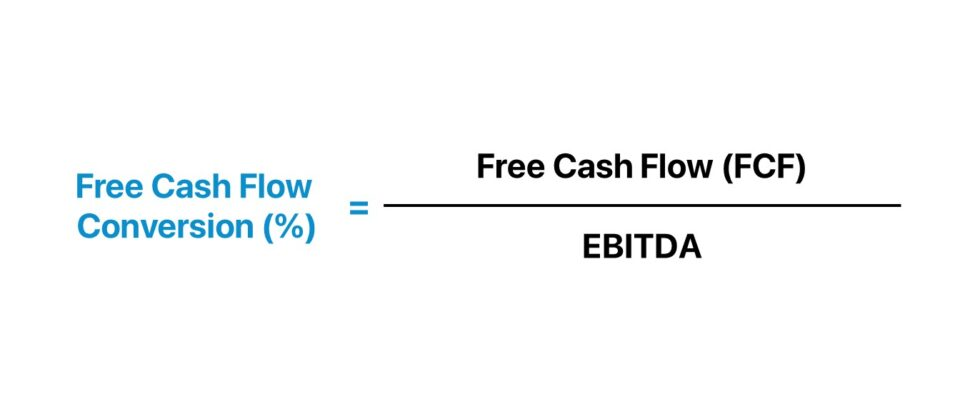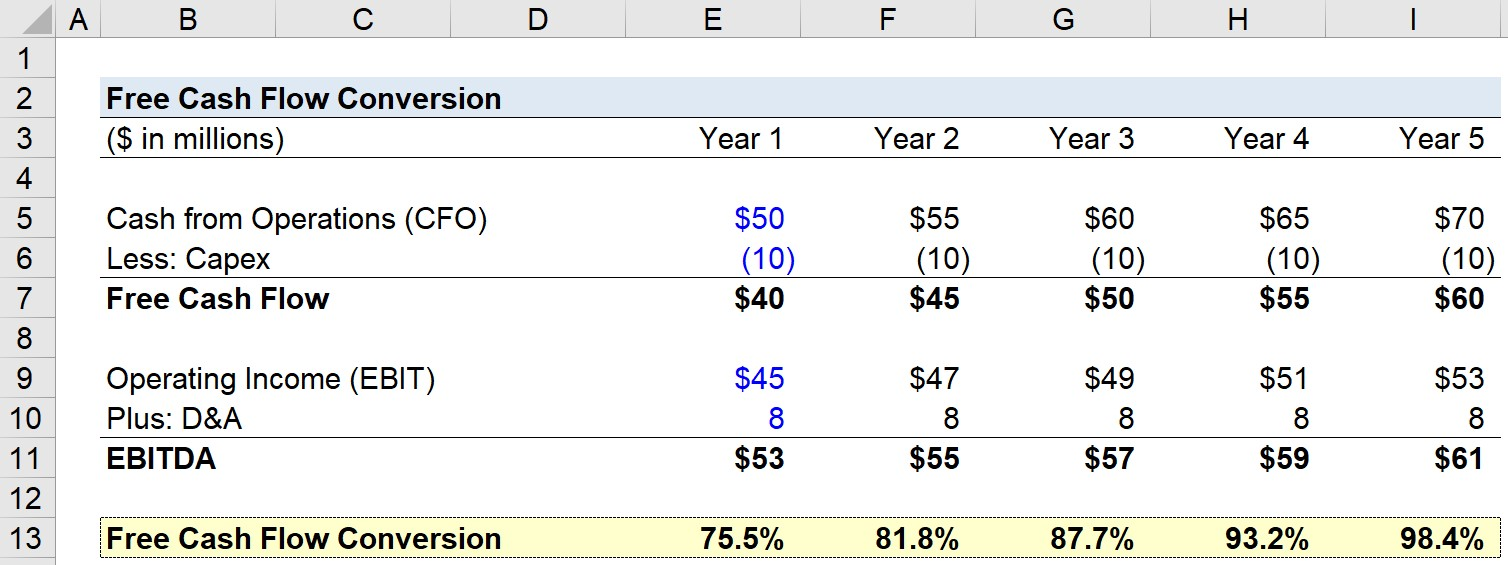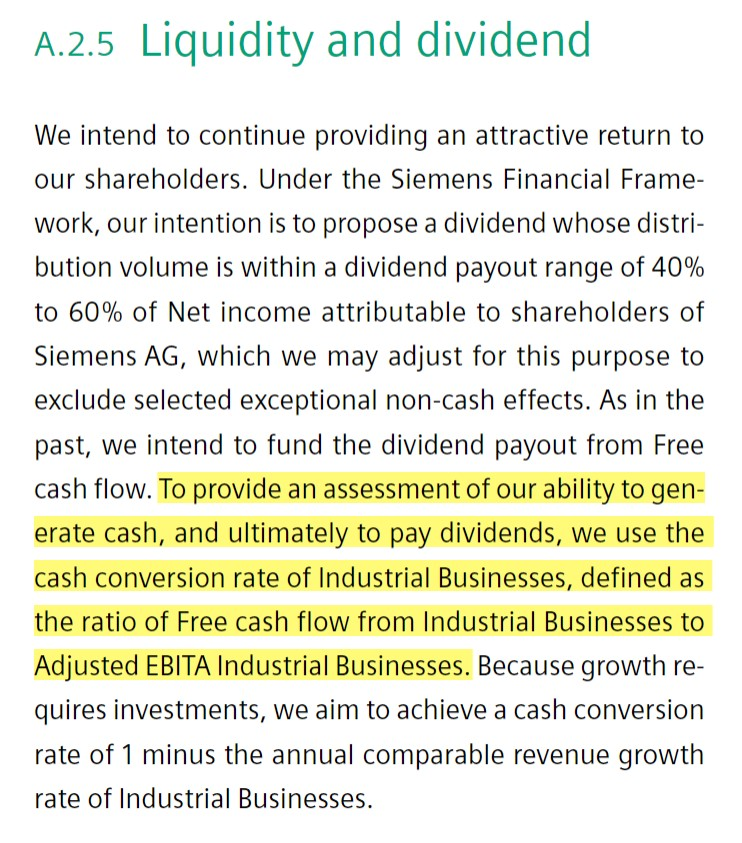Welcome to Wall Street Prep! Use code at checkout for 15% off.# Free Cash Flow Conversion (FCF)

Guide to Understanding Free Cash Flow Conversion (FCF)## How to Calculate Free Cash Flow Conversion?

The free cash flow conversion rate measures a company’s efficiency in turning its profits into free cash flow from its core operations.

The objective here is to compare a company’s free cash flow (FCF) in a given period to its EBITDA, in an effort to better understand how much FCF diverges from EBITDA.

Calculating the FCF conversion ratio comprises dividing free cash flow (FCF) by a measure of operating profitability, most often EBITDA (or EBIT).

In theory, EBITDA functions as a rough proxy for a company’s operating cash flow, albeit the metric receives much scrutiny among practitioners.

Why? The calculation of EBITDA adjusts operating income (EBIT) by adding back non-cash items, namely the depreciation and amortization (D&A) expense – which is usually the most significant non-cash expense for companies.

The issue at hand is that EBITDA neglects two major cash outflows:

1. Capital Expenditures (Capex) → The purchase of fixed assets (or PP&E).
2. Changes in Working Capital → The change in operating current assets and operating current liabilities.

To evaluate the true operating performance of a company and accurately forecast its future cash flows, these additional cash outflows and other non-cash (or non-recurring) adjustments are required to be accounted for.

## Free Cash Flow Conversion Formula (FCF)

The formula for calculating the free cash flow conversion (FCF) rate is as follows.

Free Cash Flow Conversion (FCF) = Free Cash Flow (FCF) ÷ EBITDA

Where:

• Free Cash Flow (FCF) = Cash from Operations (CFO) – Capital Expenditures (Capex)
• EBITDA = Operating Income (EBIT) + D&A

For simplicity, we’ll define free cash flow as cash from operations (CFO) minus capital expenditures (Capex).

Therefore, the FCF conversion rate can be interpreted as a company’s ability to convert its EBITDA into operating cash flow (OCF), i.e. “Cash from Operations” on the cash flow statement (CFS).

The output for the FCF conversion rate is ordinarily expressed in percentage form, but can be denoted in the form of a multiple too.

## Free Cash Flow Conversion Calculator (FCF)

We’ll now move to a modeling exercise, which you can access by filling out the form below.#### Get the Excel Template!Submitting...

## FCF Conversion Calculation Example

Suppose we’re tasked with calculating the free cash flow conversion (FCF) of a company, given the following assumptions, in Year 1.

• Cash from Operations (CFO) = \$50m
• Capital Expenditures (Capex) = \$10m
• Operating Income (EBIT) = \$45m
• Depreciation & Amortization (D&A) = \$8m

In the next step, we can calculate the free cash flow (CFO – Capex) and EBITDA:

• Free Cash Flow = \$50m CFO – \$10m Capex = \$40m
• EBITDA = \$45m EBIT + \$8m D&A = \$53m

For the rest of the forecast, we’ll be using a few more assumptions:

1. Cash from Operations (CFO) → Increasing by \$5m per year
2. Operating Income (EBIT) → Increasing by \$2m per year
3. Capex and D&A → Constant per year (i.e. “Straight-Lined”)

With these inputs, we can calculate the free cash flow conversion rate for each year, starting with Year 1.

For instance, we’ll divide the \$40m in FCF generated in Year 1 by the \$53m in EBITDA to arrive at an FCF conversion rate of 75.5%.

• Free Cash Flow Conversion (FCF) – Year 1 = \$40 million ÷ \$53 million = 75.5%

Here, we’re essentially figuring out how close a company’s discretionary free cash flow gets to its EBITDA.

In conclusion, we can see how the free cash flow conversion rate (FCF) has increased over time, from 75.5% in Year 1 to 98.4% in Year 5, which is driven by the FCF growth rate outpacing the EBITDA growth rate.## What is a Good Free Cash Flow Conversion Rate?

To perform industry comparisons, each metric should be calculated under the same standards.

In addition, management’s own calculations should be referenced, but never taken at face value and used for comparisons without first understanding which items are included or excluded.

Note that the calculation of free cash flow can be company-specific, with significant discretionary adjustments made along the way.

Often, FCF conversion rates can be most useful for internal comparisons to historical performance and to assess a company’s improvements (or lack of progress) over several time periods.Siemens Industry-Specific Cash Conversion Example (Source: 2020 10-K)

## How to Improve FCF Conversion Ratio?

A “good” free cash flow conversion rate would typically be consistently around or above 100%, as it indicates efficient working capital management.

If the FCF conversion rate of a company is in excess of 100%, that implies operational efficiency.

• Effective, Streamlined Accounts Receivables (A/R) Collection Processes
• Favorable Negotiating Terms with Suppliers
• Quicker Inventory Turnover from Increased Market Demand

In contrast, “bad” FCF conversion would be well below 100% – and can be particularly concerning if there has been a distinct pattern showing deterioration in cash flow quality year-over-year.

A sub-par FCF conversion rate suggests inefficient working capital management and potentially underperforming underlying operations, which often consists of the following operating qualities:

• Build-Up of Customer Payments made on Credit
• Tightening of Credit Terms with Suppliers
• Slowing Inventory Turnover from Lackluster Customer Demand

To reiterate from earlier, problems can easily arise because of definitions varying considerably across different companies, as most companies can adjust the formula to suit their company’s specific needs (and announced operating targets).

But as a generalization, most companies pursue a target FCF conversion rate close to or greater than 100%.Step-by-Step Online Course

### Everything You Need To Master Financial Modeling

Enroll in The Premium Package: Learn Financial Statement Modeling, DCF, M&A, LBO and Comps. The same training program used at top investment banks.